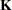The PHREG Procedure
 LSMESTIMATE Statement

LSMESTIMATE model-effect <'label'> values <divisor=>
<, <'label'> values <divisor=>> <, ...>
< / options>
;

The LSMESTIMATE statement provides a mechanism for obtaining custom hypothesis tests among least squares means.

Table 64.6 summarizes important options in the LSMESTIMATE statement. If the BAYES statement is specified, the ADJUST=, STEPDOWN, TESTVALUE, LOWER, UPPER, and JOINT options are ignored. The PLOTS= option is not available for the maximum likelihood anaysis. It is available only for the Bayesian analysis.

Table 64.6 Important LSMESTIMATE Statement Options

Option

Description

Construction and Computation of LS-Means

Modifies covariate values in computing LS-means

Computes separate margins

Specifies a list of values to divide the coefficients

Specifies the weighting scheme for LS-means computation as determined by a data set

Tunes estimability checking

Degrees of Freedom and p-values

Determines the method for multiple comparison adjustment of LS-means differences

ALPHA=Determines the confidence level ()

Performs one-sided, lower-tailed inference

Adjusts multiple comparison p-values further in a step-down fashion

TESTVALUE=

Specifies values under the null hypothesis for tests

Performs one-sided, upper-tailed inference

Statistical Output

Constructs confidence limits for means and mean differences

Displays the correlation matrix of LS-means

Displays the covariance matrix of LS-means

Prints thematrix

Prints thematrix

Produces a jointor chi-square test for the LS-means and LS-means differences

Requests ODS statistical graphics of means and mean comparisons

Specifies the seed for computations that depend on random numbers

For details about the syntax of the LSMESTIMATE statement, see the section LSMESTIMATE Statement of Chapter 19, Shared Concepts and Topics.Previous Page | Next Page | Top of Page